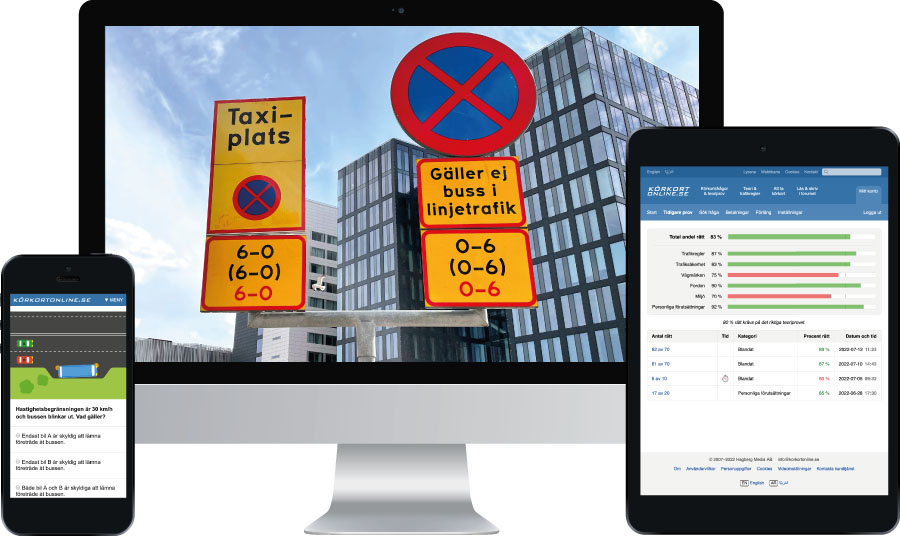# Driving theory questions

If you are driving at 100 km/h and increase your speed to 110 km/h, how much time do you gain per 10 km?

The options are not shown, since this is one of the 1000 paid questions.

Driving Licence Book (19th Edition, page 84):
If you increase your average speed by 10 km/h, the time gain per 10 km will be:
– approximately 1 minute at speeds under 90 km/h
– approximately ½ minute at speeds over 90 km/h

In all likelihood, you will not need to know the following mathematical calculations for the real test. It is enough to know that the time gain is minimal.

## Method 1

 Colour codes for the figures (easier to keep track of them) Original speed 100 km/h New speed 110 km/h Minutes per hour (since the speed is km/h, kilometres per hour) 60 minutes Number of kilometres 10 km

We first calculate how many minutes it takes to travel 1 km at both speeds:

• 60 / 100 = it takes 0.6 minutes to travel 1 km
• 60 / 110 = it takes 0.54 minutes to travel 1 km

It is therefore takes slightly less time with the higher speed. The difference is:

• 0.6 - 0.54 = 0.06 minutes faster per km when travelling at 110 km/h compared with 100 km/h.

However, the question is how much time you gain per 10 km, not per km. Therefore, recalculate the time gain per 10 km:

• 0.06 * 10 = 0.6 minutes time gain per 10 km

Now it is really done. It is however easier to understand if the answer is recalculated to seconds:

• 0.6 * 60 = 36 seconds

## Method 2

 Colour codes for the figures (easier to keep track of them) Original speed 100 km/h New speed 110 km/h Distance 10 km = 10,000 metres Fixed conversion rate km/h to m/s 3.6 times

Formula for calculation of time:

• Distance / speed = time

For the formula to work requires us to use metres instead of 10 km and metres per second (m/s) instead of kilometres an hour (km/h). The speeds are therefore recalculated to m/s:

• 100 / 3.6 = 27.78 m/s
• 110 / 3.6 = 30.56 m/s

Only now can we use the formula Distance / speed = time:

• 10,000 / 27.78 = 360 s
• 10,000 / 30.56 = 327 s

We can then work out the time difference between both speeds:

• 360 - 327 = 33 seconds

This is an example of one of the driving theory questions at Körtkortonline.se (also in theory tests in Arabic).

There is also a driving licence book online (2023).

More examples of theory questions:

Category:

## Buy all 1000 questions in EnglishPersonal account with all 1000 questions.

Unlimited tests during the validity period.

Choose validity period: